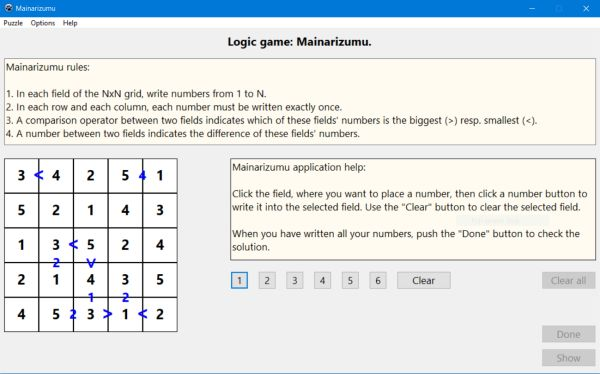# Computing: Free Pascal Programming

## Logic number puzzle: Mainarizumu.

Description: Mainarizumu (also called Minarism) is a puzzle game with numbers to be placed onto a grid. It may be viewed as a variant of Futoshiki (with no numbers displayed at game begin). The rules of the game are as follows:
1. In each field of the NxN grid, write numbers from 1 to N.
2. In each row and each column, each number must be written exactly once.
3. A comparison operator between two fields indicates which of these fields' numbers is the biggest (>) resp. smallest (<).
4. A number between two fields indicates the difference of these fields' numbers.
The game is a typical logic game: In order to find the correct solution of the puzzle, you have to explore the numbers already written, and by logical thinking (concluding from what you know, that a given field must be a given number, or on the contrary, can't be a given number), determine, which fields have to contain which numbers.
The game is played with the mouse: Click the field, where you want to write a number, then click one of the number buttons to write the corresponding number or Clear to remove the number from the actually selected field.

Free Pascal features: Creating objects during run-time. Changing the color of shapes and the caption and font of static-texts during run-time. Using buttons to insert characters into a given control's caption. Two-dimensional arrays, arrays of records (classic Pascal).

Screenshot: